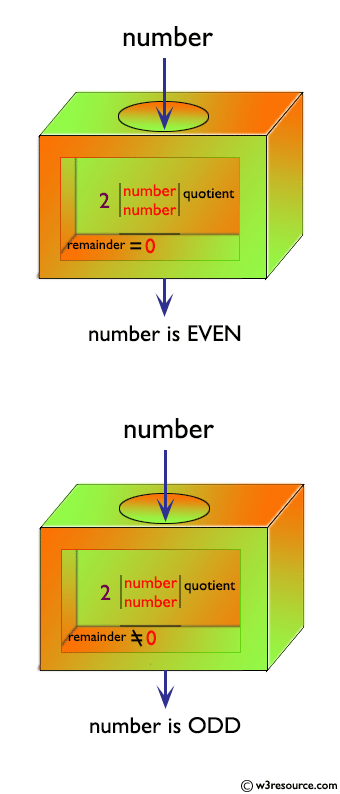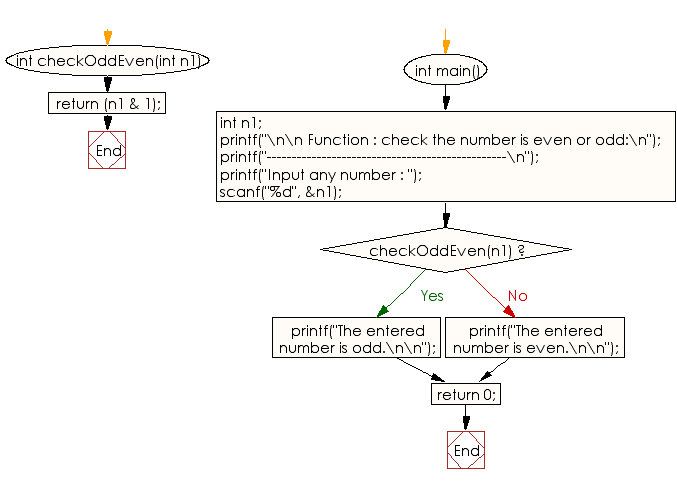﻿ C Program: Check the given number is even or odd - w3resource

# C Exercises: Check the given number is even or odd

## C Function : Exercise-4 with Solution

Write a program in C to check if a given number is even or odd using the function.

Pictorial Presentation:Sample Solution:

C Code:

``````#include <stdio.h>

//if the least significant bit is 1 the number is odd and 0 the number is even
int checkOddEven(int n1)
{
return (n1 & 1);//The & operator does a bitwise and,
}

int main()
{
int n1;
printf("\n\n Function : check the number is even or odd:\n");
printf("------------------------------------------------\n");
printf("Input any number : ");
scanf("%d", &n1);

// If checkOddEven() function returns 1 then the number is odd
if(checkOddEven(n1))
{
printf("The entered number is odd.\n\n");
}
else
{
printf("The entered number is even.\n\n");
}
return 0;
}
```
```

Sample Output:

``` Function : check the number is even or odd:
------------------------------------------------
Input any number : 5
The entered number is odd.
```

Explanation:

```int checkOddEven(int n1) {
return (n1 & 1); //The & operator does a bitwise and,
}
```

The above function 'checkOddEven' takes a single argument of type int, named 'n1'. It checks whether the value of 'n1' is odd or even by performing a bitwise AND operation between 'n1' and the binary value 1.

If the result of this operation is 1, then the least significant bit of n1 is 1, which means that n1 is odd.

If the result is 0, then the least significant bit of n1 is 0, which means that n1 is even. The function then returns the result of as an integer value of 0 (even) or 1 (odd).

Time complexity and space complexity:

The time complexity of the function ‘checkOddEven’ is O(1), as the computation it performs is constant and independent of the input size.

The space complexity of this function is also O(1), as it only uses a fixed amount of memory to store the single integer variable n1.

Flowchart:C Programming Code Editor:

What is the difficulty level of this exercise?

Test your Programming skills with w3resource's quiz.

﻿